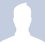# Between imaginary index and imaginary power

With i = $\sqrt{-1}$,

Let say i = $e^{\frac{\pi}{2} i}$.

[$\displaystyle e^{\frac{\pi}{2} i}$]$^i$ = [$\displaystyle e^{\frac{\pi}{2} i}$]$^\frac{i^2}{i}$, {Index verses Power}

The whole thing of $i i$ or $i \frac{i^2}{i}$ concerning only index and power makes -1 as a real number.

However, | z | = | i | = 1 of Ln | z | = 0 can be said as a case at critical intermediate.

Where [$\displaystyle e^{\frac{\pi}{2} i}$]$^\frac{1}{i}$ = [$\displaystyle e^{\frac{\pi}{2} i}$]$^\frac{i}{i^2}$ can have a genuine cancellation between numerator and denominator,

$i i$ or $i \frac{i^2}{i}$ is considered as multiplication between i and i to mix up into real part of the index for a broken value.

To take golden wing of 1 to hold true at critical point, [$\displaystyle e^{\frac{\pi}{2} i}$]$^\frac{1}{i}$ = $e^ \frac{\pi}{2}$ = 4.8104773809653516554730356667038+ ought to be taken as true rather than a broken range.

Slightly illogical at critical point! This explains why there seem to be contradiction to occur for $1^1 = 2^1 = 3^1 = \ldots$ with real power of 1 via complex realm. However, golden wing simplified into a real number of 1 while the formation is form of an $\frac{i}{i}$ rather than an $i i$.

The simple thing that can be stated is imaginary index and complex indices are valid while imaginary power and complex powers to any given numbers are mostly invalid, unless they are real resultant wings going through $\frac{i}{i}$ rather than $i i$.

It is true that $\frac{a + b \sqrt{-1}}{c + d \sqrt{-1}}$ can always become simply $e + f \sqrt{-1}$, however, the main thing for a valid case is for no mixing up between Ln | z | part and $\sqrt{-1}$ Arg z part, when a power is taken by any number consists of complex indices in general.

$1^1 = 2^1 = 3^1 = \ldots$ is something optional perhaps which is up to you onto how you would think about them. Nevertheless, there is nothing risky which goes against arithmetic of General Number.

We extend critical cases into general cases: Complex indices are valid and complex powers are mostly invalid (or invalid if you like). I personally take both General Number and Golden wing of 1 to be true. This is most beneficial to me.

As a conclusion, we don't find trouble for a given power to general number. Power of complex numbers and above are just invalid#.Note by Lu Chee Ket
5 years, 7 months ago

This discussion board is a place to discuss our Daily Challenges and the math and science related to those challenges. Explanations are more than just a solution — they should explain the steps and thinking strategies that you used to obtain the solution. Comments should further the discussion of math and science.

When posting on Brilliant:

• Use the emojis to react to an explanation, whether you're congratulating a job well done , or just really confused .
• Ask specific questions about the challenge or the steps in somebody's explanation. Well-posed questions can add a lot to the discussion, but posting "I don't understand!" doesn't help anyone.
• Try to contribute something new to the discussion, whether it is an extension, generalization or other idea related to the challenge.
• Stay on topic — we're all here to learn more about math and science, not to hear about your favorite get-rich-quick scheme or current world events.

MarkdownAppears as
*italics* or _italics_ italics
**bold** or __bold__ bold
- bulleted- list
• bulleted
• list
1. numbered2. list
1. numbered
2. list
Note: you must add a full line of space before and after lists for them to show up correctly
paragraph 1paragraph 2

paragraph 1

paragraph 2

[example link](https://brilliant.org)example link
> This is a quote
This is a quote
    # I indented these lines
# 4 spaces, and now they show
# up as a code block.

print "hello world"
# I indented these lines
# 4 spaces, and now they show
# up as a code block.

print "hello world"
MathAppears as
Remember to wrap math in $$ ... $$ or $ ... $ to ensure proper formatting.
2 \times 3 $2 \times 3$
2^{34} $2^{34}$
a_{i-1} $a_{i-1}$
\frac{2}{3} $\frac{2}{3}$
\sqrt{2} $\sqrt{2}$
\sum_{i=1}^3 $\sum_{i=1}^3$
\sin \theta $\sin \theta$
\boxed{123} $\boxed{123}$

## Comments

There are no comments in this discussion.

×

Problem Loading...

Note Loading...

Set Loading...